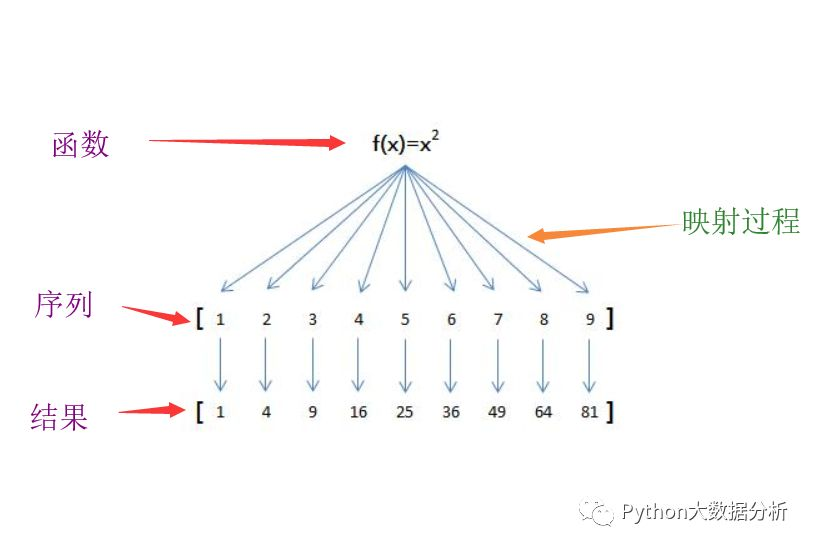# 如何分析python的map、reduce函数01

map

map()方法会将 一个函数 映射序列的每一个元素上，生成新序列，包含所有函数返回值。```

map(function_to_apply, list_of_inputs)```
• function_to_apply：代表函数

• list_of_inputs：代表输入序列

```

items = [1, 2, 3, 4, 5] # 列表

squared = []

for i in items:

squared.append(i**2)```

map函数可以让我们用一种简单而漂亮得多的方式来实现：

```

items = [1, 2, 3, 4, 5]

squared = list(map(lambda x: x**2, items))```

```

items = [1, 2, 3, 4, 5]

def f(x):

return x**2

squared = list(map(f, items))```

02

reduce

reduce相比map稍复杂点

reduce的工作过程是 ：在迭代序列的过程中，首先把 前两个元素（只能两个）传给 函数，函数加工后，然后把 得到的结果和第三个元素 作为两个参数传给函数参数， 函数加工后得到的结果又和第四个元素 作为两个参数传给函数参数，依次类推。reduce函数怎么用？

```

reduce(function, iterable[, initializer])```
• function：代表函数

• iterable：序列

• initializer：初始值（可选）```

# 导入reduce

from functools import reduce

# 定义函数

def f(x,y):

return x*y

# 定义序列，含1~10的元素

items = range(1,11)

# 使用reduce方法

result = reduce(f,items)

print(result)```